Latest Teaching jobs   »   Reasoning Questions For DSSSB/HSSC Exam |...

# Reasoning Questions For DSSSB/HSSC Exam | 3rd October 2019The reasoning is the most scoring section in any competitive exam. It also develops or nourishes our critical thinking skills as compared to other subjects. In DSSSBKVSAPS Exam as a student to score well in this part, you have to start your preparation now because there is negative marking as well. The more you have prepared, the better you score.

Directions(Q1-2): In each of the following questions, choose a word from the given alternatives, that is similar to the given words and hence belongs to the same group.

Q1. Geetanjali: Tagore
(a) Arthashastra: Tulsidas
(b) Shakuntla: Kautilya
(c) Das Capital: Hitler
(d) Oliver Twist: Dicken

Q2. Murder: Crime
(b) Chapter: Book
(c) Lie: Falsehood
(d) Sonnet: Poem

Q3. In the certain code RAID is written as % # * \$, RIPE is written as % * @!. How is DEAR written up in that code?
(a) @!#%
(b) \$@#%
(c) @\$#%
(d) \$!#%

Q4. In a certain code MODERN is written as 5%38#@ and WIN is written as 6!@. How is RIDE written in that code?
(a) #!38
(b) #@38
(c) \$!83
(d) !@38

Q5. In a certain code ROPE is written as %57\$, DOUBT is written as 35#8* and LIVE is written as @24\$. How is TROUBLE written in that code?
(a) *%5#8@\$
(b) *%#58@\$
(c) *%5#8@4
(d) *%#58\$@

Q6. If + means –, – means ×, ÷ means + and × means ÷, then 15 – 3 + 10 × 5 ÷ 5 =?
(a) 5
(b) 22
(c) 48
(d) 52

Q7. If P means ‘division’, T means ‘addition’, M means ‘subtraction’ and D means ‘multiplication’, then what will be the value of the expression 12 M12 D 28 P 7 T 15 ?
(a) –30
(b) –21
(c) 15
(d) 45

Q8. In the given below how many paths are there from A to X if the only ways to move are up and to the right?(a) 8
(b) 9
(c) 3
(d) 6

Q9. 0, 6, 24, 60, 120, 210,?
(a) 343
(b) 336
(c) 295
(d) None of these

Q10. 5, 6,?, 57, 244
(a) 15
(b) 16
(c) 17
(d) None of these

Solutions

S1. Ans.(d)
Sol. ‘Geetanjali’ is written by ‘Tagore’.
Similarly, ‘Oliver Twist’ is written by ‘Dicken’.

S2. Ans.(d)
Sol. ‘Murder’ is a type of ‘Crime’.
Similarly, ‘Sonnet’ is a type of ‘Poem’.
‘Sonnet’ is a poem that has 14 lines, each containing 10 syllables and a fixed pattern of Rhyme.

S3. Ans.(d)
Sol. According to the questionsTherefore,S4. Ans.(a)
Sol. According to the questionTherefore,S5. Ans.(a)
Sol. According to the questionTherefore,S6. Ans.(c)
Sol. The given expression is 15 – 3 + 10 × 5 ÷ 5
Using the symbols correctly as given in the above question, the above expression becomes
15 × 3 – 10 ÷ 5 + 5
Using BODMAS RULE, we get
15 × 3 – 2 + 5 = 45 – 2 + 5 = 50 – 2 = 48
Therefore, the value of the given expression = 48

S7. Ans.(b)
Sol. The given expression is 12 M 12 D 28 P 7 T 15
Using the symbols correctly as given in the above question, the above expression becomes
12 – 12 × 28 ÷ 7 + 15
Using BODMAS RULE, we get
12 – 12 × 4 + 15 = 12 – 48 + 15 = 27 – 48 = –21
Therefore, the value of the given expression = –21

S8. Ans.(d)
Sol. In the given figure, label all the points of intersection
So, we getTherefore, there are six such ways from A to X namely ABCDX, ABEDX, ABEHX, AFEDX, AFEHX, AFGHX.

S9. Ans.(b)
Sol. The given sequence of number follows the pattern:Therefore, Missing number = 210 + 126 = 336.

S10. Ans.(b)
Sol. The given sequence of number follows the pattern:Therefore, Missing number = 6 × 2 + 2² = 16.

You may also like to read :

Sharing is caring!

### TOPICS:

•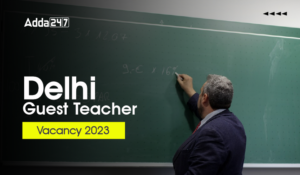Delhi Guest Teacher Vacancy 2023 For PRT...
•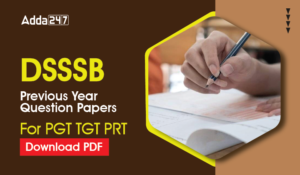DSSSB Previous Year Question Paper For P...
•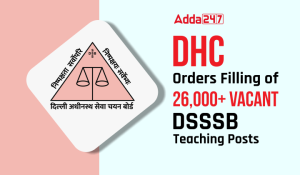DHC Orders Filling of 26,000 Vacant DSSS...
•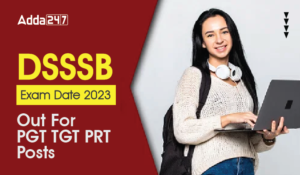DSSSB PGT Exam Date 2023 Out, Check Shif...
•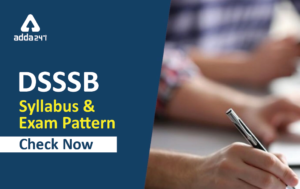DSSSB Syllabus 2023 For TGT PGT PRT Down...
•4500+ DSSSB TGT Vacancies Expected to be...